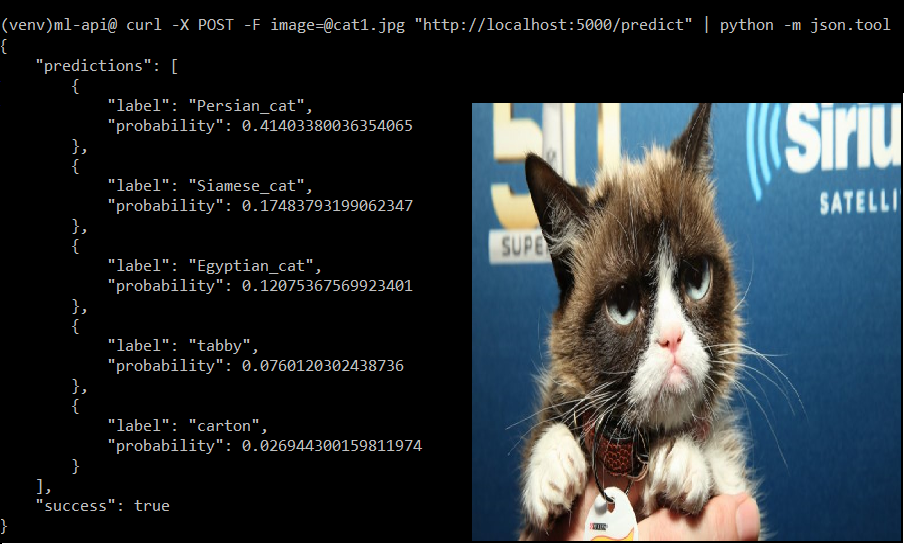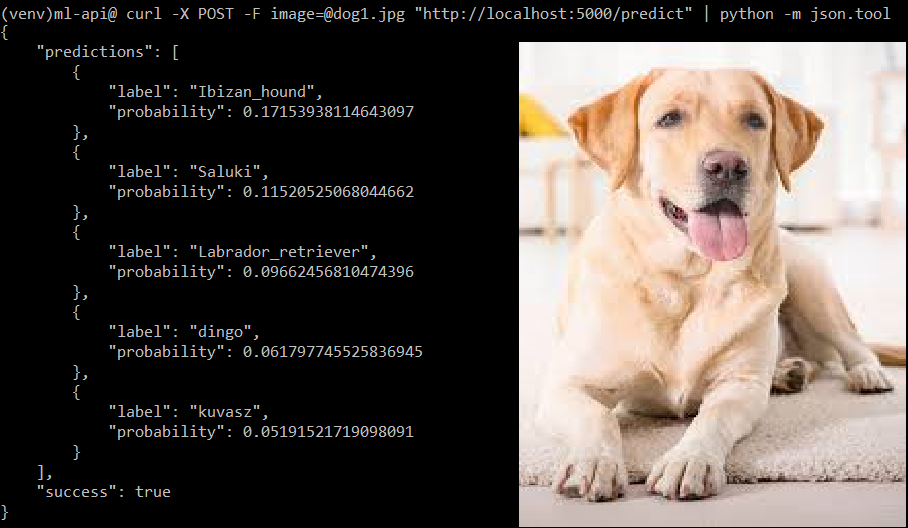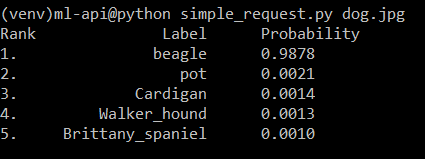# Exposing ML/DL Models as REST APIs

• Difficulty Level : Medium
• Last Updated : 17 Jan, 2019

Frameworks we’ll be using:
Keras is a Deep Learning library, built on top of backends such as Tensorflow, Theano or CNTK. It provides abstraction and allows rapid development of ML/DL models.

Flask is a micro web framework in Python, which is used to quickly spin up servers to serve pages. Refer Introduction to Flask.

Since the focus of the article is to serve a ML/DL model using an API, we wouldn’t get deeper into the making of the Convolutional model. We will be using the ResNet50 Convolutional Neural Network.

Install Tensorflow and Keras

StepLinux/MacWindows
Update pippip install -U pippython -m pip install -U pip
Install virtualenvsudo pip install virtualenvpip install virtualenv
Create a new folder ml-apimkdir ml-api && cd ml-apimkdir ml-api && cd ml-api
Create a virtual environment(Good practice) and activate itvirtualenv –system-site-packages -p python3 ./venvvirtualenv –system-site-packages -p python3 ./venv
Activate the virtual environmentsource ./venv/bin/activate

.\venv\Scripts\activate
Install Keraspip install keraspip install keras
Install other dependenciespip install flask gevent requests pillowpip install flask gevent requests pillow

Examples:Creating the REST API :

 `# keras_server.py`` ` `# Python program to expose a ML model as flask REST API`` ` `# import the necessary modules``from` `keras.applications ``import` `ResNet50 ``# pre-built CNN Model``from` `keras.preprocessing.image ``import` `img_to_array ``from` `keras.applications ``import` `imagenet_utils``import` `tensorflow as tf``from` `PIL ``import` `Image``import` `numpy as np``import` `flask``import` `io`` ` `# Create Flask application and initialize Keras model``app ``=` `flask.Flask(__name__)``model ``=` `None`` ` `# Function to Load the model ``def` `load_model():``     ` `    ``# global variables, to be used in another function``    ``global` `model     ``    ``model ``=` `ResNet50(weights ``=``"imagenet"``)``    ``global` `graph ``    ``graph ``=` `tf.get_default_graph()`` ` `# Every ML/DL model has a specific format``# of taking input. Before we can predict on``# the input image, we first need to preprocess it.``def` `prepare_image(image, target):``    ``if` `image.mode !``=` `"RGB"``:``        ``image ``=` `image.convert(``"RGB"``)``     ` `    ``# Resize the image to the target dimensions``    ``image ``=` `image.resize(target) ``     ` `    ``# PIL Image to Numpy array``    ``image ``=` `img_to_array(image) ``     ` `    ``# Expand the shape of an array,``    ``# as required by the Model``    ``image ``=` `np.expand_dims(image, axis ``=` `0``) ``     ` `    ``# preprocess_input function is meant to``    ``# adequate your image to the format the model requires``    ``image ``=` `imagenet_utils.preprocess_input(image) `` ` `    ``# return the processed image``    ``return` `image`` ` `# Now, we can predict the results.``@app``.route(``"/predict"``, methods ``=``[``"POST"``])``def` `predict():``    ``data ``=` `{} ``# dictionary to store result``    ``data[``"success"``] ``=` `False`` ` `    ``# Check if image was properly sent to our endpoint``    ``if` `flask.request.method ``=``=` `"POST"``:``        ``if` `flask.request.files.get(``"image"``):``            ``image ``=` `flask.request.files[``"image"``].read()``            ``image ``=` `Image.``open``(io.BytesIO(image))`` ` `            ``# Resize it to 224x224 pixels ``            ``# (required input dimensions for ResNet)``            ``image ``=` `prepare_image(image, target ``=``(``224``, ``224``))`` ` `        ``# Predict ! global preds, results``            ``with graph.as_default():``                ``preds ``=` `model.predict(image)``                ``results ``=` `imagenet_utils.decode_predictions(preds)``                ``data[``"predictions"``] ``=` `[]`` ` `         ` `            ``for` `(``ID``, label, probability) ``in` `results[``0``]:``                ``r ``=` `{``"label"``: label, ``"probability"``: ``float``(probability)}``                ``data[``"predictions"``].append(r)`` ` `            ``data[``"success"``] ``=` `True`` ` `    ``# return JSON response``    ``return` `flask.jsonify(data)`` ` ` ` ` ` `if` `__name__ ``=``=` `"__main__"``:``    ``print``((``"* Loading Keras model and Flask starting server..."``        ``"please wait until server has fully started"``))``    ``load_model()``    ``app.run()`

`python keras_server.py `

Note #1: For the first time you run it, it takes time to download the weights of the model. In subsequent runs, this won’t be the case.
Note #2: Save images in the same directory (ml-api) – dog.jpg, cat.jpg, lion.jpg .

### Make requests to the server:

`\$ curl -X POST -F image=@dog.jpg "http://localhost:5000/predict"`

Method #2 : (Suitable if making a Full-stack web app with proper UI)
Create a simple HTML form

 ```<``form` `action` `= ``"http://localhost:5000/predict"` `method` `= ``"POST"` `         ``enctype` `= ``"multipart/form-data"``>``         ``<``input` `type` `= ``"file"` `name` `= ``"image"` `/>``         ``<``input` `type` `= ``"submit"``/>```

Method #3 : Create a simple python script to make HTTP requests to flask server.

 `# simple_request.py``import` `requests``import` `sys`` ` `URL ``=` `"http://localhost:5000/predict"`` ` `# provide image name as command line argument``IMAGE_PATH ``=` `sys.argv[``1``] `` ` `image ``=` `open``(IMAGE_PATH, ``"rb"``).read()``payload ``=` `{``"image"``: image}`` ` `# make request to the API``request ``=` `requests.post(URL, files ``=` `payload).json()`` ` `if` `request[``"success"``]:``    ``# Print formatted Result``    ``print``(``"% s % 15s % s"``%``(``"Rank"``, ``"Label"``, ``"Probability"``))``    ``for` `(i, result) ``in` `enumerate``(request[``"predictions"``]):``        ``print``(``"% d.    % 17s %.4f"``%``(i ``+` `1``, result[``"label"``],``            ``result[``"probability"``]))`` ` `    ``else``:``        ``print``(``"Request failed"``)`

Run it as :

`python simple_request.py dog.jpg`

Output:To deactivate after you are done(both Windows and Linux) :

```> deactivate
```

So the workflow, in general for any model is

• Build the model and save it.